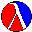# Section 13Intermezzo 2: List AbbreviationsIntermediate Student

Using `cons` to create lists is cumbersome if a list contains many items. Fortunately, Scheme provides the `list` operation, which consumes an arbitrary number of values and creates a list. Here is Scheme's extended syntax:

```<prm> = list
```

The extended collection of values is

```<val> = (list <val> ... <val>)
```

A simpler way to understand `list` expressions is to think of them as abbreviations. Specifically, every expression of the shape

```(list exp-1 ... exp-n)
```

stands for a series of n `cons` expressions:

```(cons exp-1 (cons ... (cons exp-n empty)))
```

Recall that `empty` is not an item of the list here, but the rest of the list. Here are three examples:

```(list 1 2)
= (cons 1 (cons 2 empty))

(list 'Houston 'Dallas 'SanAntonio)
= (cons 'Houston (cons 'Dallas (cons 'SanAntonio empty)))

(list false true false false)
= (cons false (cons true (cons false (cons false empty))))
```

They introduce lists with two, three, and four items, respectively.

Of course, we can apply `list` not only to values but also to expressions:

```  (list (+ 0 1) (+ 1 1))
= (list 1 2)
```

Before the list is constructed, the expressions must be evaluated. If during the evaluation of an expression an error occurs, the list is never formed:

```  (list (/ 1 0) (+ 1 1))
= /: divide by zero
```

In short, `list` behaves just like any other primitive operation.

The use of `list` greatly simplifies the notation for lists with many items and lists that contains lists or structures. Here is an example:

```  (list 0 1 2 3 4 5 6 7 8 9)
```

This list contains 10 items and its formation with `cons` and `empty` would require 10 uses of `cons` and one instance of `empty`. Similarly, the list

```  (list (list 'bob 0 'a)
(list 'carl 1 'a)
(list 'dana 2 'b)
(list 'erik 3 'c)
(list 'frank 4 'a)
(list 'grant 5 'b)
(list 'hank 6 'c)
(list 'ian 8 'a)
(list 'john 7 'd)
(list 'karel 9 'e))
```

requires 11 uses of `list` in contrast to 40 of `cons` and 11 of `empty`.

Exercise 13.0.3.   Use `cons` and `empty` to construct the equivalent of the following lists:

1. `(list 0 1 2 3 4 5)`

2. `(list (list 'adam 0) (list 'eve 1) (list 'louisXIV 2))`

3. `(list 1 (list 1 2) (list 1 2 3))`.Solution

Exercise 13.0.4.   Use `list` to construct the equivalent of the following lists:

1. `(cons 'a (cons 'b (cons 'c (cons 'd (cons 'e empty)))))`

2. `(cons (cons 1 (cons 2 empty)) empty)`

3. `(cons 'a (cons (cons 1 empty) (cons false empty)))`.

4. `(cons (cons 1 (cons 2 empty)) (cons (cons 2 (cons 3 empty)) empty))`

Start by determining how many items each list and each nested list contains.Solution

Exercise 13.0.5.   On rare occasions, we encounter lists formed with `cons` and `list`. Reformulate the following lists using `cons` and `empty` exclusively:

1. `(cons 'a (list 0 false))`

2. `(list (cons 1 (cons 13 empty)))`

3. `(list empty empty (cons 1 empty))`

4. `(cons 'a (cons (list 1) (list false empty)))`.

Then formulate the lists using `list`.Solution

Exercise 13.0.6.   Determine the values of the following expressions:

1. `(list (symbol=? 'a 'b) (symbol=? 'c 'c) false)`

2. `(list (+ 10 20) (* 10 20) (/ 10 20))`

3. `(list 'dana 'jane 'mary 'laura)`Solution

Exercise 13.0.7.   Determine the values of

```(first (list 1 2 3))

(rest (list 1 2 3))
```Solution

The use of `list` makes it significantly easier to evaluate expressions involving lists. Here are the recursive steps from an example from section 9.5:

```  (sum (list (make-ir 'robot 22.05) (make-ir 'doll 17.95)))
= (+ (ir-price (first (list (make-ir 'robot 22.05) (make-ir 'doll 17.95))))
(sum (rest (list (make-ir 'robot 22.05) (make-ir 'doll 17.95)))))
= (+ (ir-price (make-ir 'robot 22.05))
(sum (list (make-ir 'doll 17.95))))
At this place, we use one of the equations governing
the new primitive operations for the first time:
= (+ 22.05
(sum (list (make-ir 'doll 17.95))))
= (+ 22.05
(+ (ir-price (first (list (make-ir 'doll 17.95))))
(sum (rest (list (make-ir 'doll 17.95))))))
= (+ 22.05
(+ (ir-price (make-ir 'doll 17.95))
(sum empty)))
= (+ 22.05 (+ 17.95 (sum empty)))
= (+ 22.05 (+ 17.95 0))
```

Since the laws of `first` and `rest` carry over to `list` values in a natural manner, an evaluation using `list` does not need to expand `list` into uses of `cons` and `empty`.

Following an old programming language convention,39 we may abbreviate lists and symbols even further. If a list is formulated with `list`, we can simply agree to drop list and that each opening parenthesis stands for itself and the word `list`. For example,

```'(1 2 3)
abbreviates
(list 1 2 3)
or
(cons 1 (cons 2 (cons 3 empty))) .
```

Similarly,

```'((1 2) (3 4) (5 6))
stands for
(list (list 1 2) (list 3 4) (list 5 6)),
```

which can be further expanded into `cons` and `empty` expressions.

If we drop quotes in front of symbols, writing lists of symbols is a breeze:

```'(a b c)
This short-hand is an abbreviation for
(list 'a 'b 'c)
```

And, more impressively,

```'(<html>
(<title> My First Web Page)
(<body> Oh!))
stands for
(list '<html>
(list '<title> 'My 'First 'Web 'Page)
(list '<body> 'Oh!)) .
```

Exercise 13.0.8.   Restore `list` and quotes where necessary:

1.

```'(1 a 2 b 3 c)
```

2.

```'((alan 1000)
(barb 2000)
(carl 1500)
(dawn 2300))
```

3.

```'((My First Paper)
(Sean Fisler)
(Section 1
(Subsection 1 Life is difficult)
(Subsection 2 But learning things makes it interesting))
(Section 2
Conclusion? What conclusion?))
```Solution

39 The convention is due to LISP, an early but highly advanced programming language designed in 1958. Scheme inherited many ideas from LISP, but it is a different language.